A potentiometer is used for the comparison of e.m.f. of two cells E1 and E2. For cell E1 the no deflection point is obtained at 20cm and for E2 the no deflection point is obtained at 30cm. The ratio of their e.m.f.'s will be

(1) 2/3

(2) 1/2

(3) 1

(4) 2

Concept Questions :-

Meter bridge and potentiometer
High Yielding Test Series + Question Bank - NEET 2020

Difficulty Level:

If the length of the potentiometer wire is increased, then the length of the previously obtained balance point will :

(1) Increase

(2) Decrease

(3) Remain unchanged

(4) Become two times

Concept Questions :-

Meter bridge and potentiometer
High Yielding Test Series + Question Bank - NEET 2020

Difficulty Level:

If in the experiment of Wheatstone's bridge, the positions of cells and galvanometer are interchanged, then balance points will

(1) Change

(2) Remain unchanged

(3) Depend on the internal resistance of cell and resistance of galvanometer

(4) None of these

Concept Questions :-

Wheatstone bridge
High Yielding Test Series + Question Bank - NEET 2020

Difficulty Level:

Two cells when connected in series are balanced on 8m on a potentiometer. If the cells are connected with polarities of one of the cells  reversed, they balance on 2m. The ratio of e.m.f.'s of the two cells is

(1) 3 : 5

(2) 5 : 3

(3) 3 : 4

(4) 4 : 3

Concept Questions :-

Grouping of cells
High Yielding Test Series + Question Bank - NEET 2020

Difficulty Level:

In the diagram shown, the reading of voltmeter is 20 V and that of ammeter is 4 A. The value of R should be (consider given ammeter and voltmeter are not ideal) :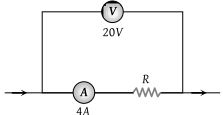(1) Equal to 5 Ω

(2) Greater from 5 Ω

(3) Less than 5 Ω

(4) Greater or less than 5 Ω depends on the material of R

Concept Questions :-

Kirchoff's voltage law
High Yielding Test Series + Question Bank - NEET 2020

Difficulty Level:

Which is a wrong statement :

(1) The Wheatstone bridge is most sensitive when all the four resistances are of the same order

(2) In a balanced Wheatstone bridge, interchanging the positions of galvanometer and cell affects the balance of the bridge

(3) Kirchhoff's first law (for currents meeting at a junction in an electric circuit) expresses the conservation of charge

(4) The rheostat can be used as a potential divider

Concept Questions :-

Wheatstone bridge
High Yielding Test Series + Question Bank - NEET 2020

Difficulty Level:

AB is a wire of uniform resistance. The galvanometer G shows no current when the length AC = 20cm and CB = 80 cm. The resistance R is equal to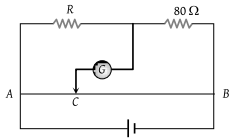(1) 2 Ω

(2) 8 Ω

(3) 20 Ω

(4) 40 Ω

Concept Questions :-

Meter bridge and potentiometer
High Yielding Test Series + Question Bank - NEET 2020

Difficulty Level:

The circuit shown here is used to compare the e.m.f. of two cells ${E}_{1}$ and ${E}_{2}\left({E}_{1}>{E}_{2}\right)$. The null point is at C when the galvanometer is connected to E1. When the galvanometer is connected to E2, the null point will be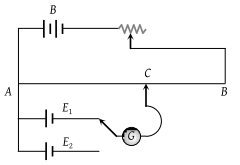(1) To the left of C

(2) To the right of C

(3) At C itself

(4) Nowhere on AB

Concept Questions :-

Meter bridge and potentiometer
High Yielding Test Series + Question Bank - NEET 2020

Difficulty Level:

In the Wheatstone's bridge (shown in figure) X = Y and A > B. The direction of the current between ab will be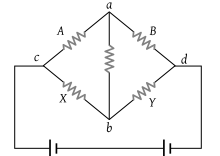(1) From a to b

(2) From b to a

(3) From b to a through c

(4) From a to b through c

Concept Questions :-

Wheatstone bridge
High Yielding Test Series + Question Bank - NEET 2020

Difficulty Level:

A resistance of 4 Ω and a wire of length 5 metres and resistance 5 Ω are joined in series and connected to a cell of e.m.f. 10 V and internal resistance 1 Ω. A parallel combination of two identical cells is balanced across 300 cm of the wire. The e.m.f. E of each cell is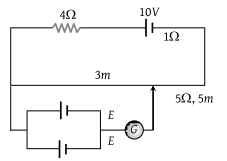(1) 1.5 V

(2) 3.0 V

(3) 0.67 V

(4) 1.33 V

Concept Questions :-

Meter bridge and potentiometer